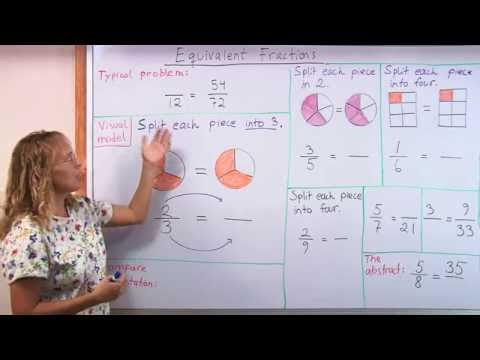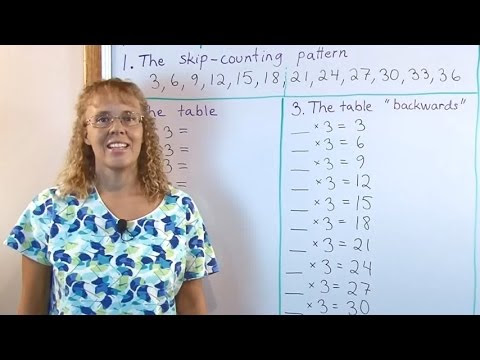## Posts

Showing posts from February, 2009

### Equivalent fractions - a visual model of splitting the pieces furtherWith learning fractions, there is always the problem of "so many rules to remember". I offer this visual method of splitting the pieces further, and using the arrow notation as a remedy; hopefully this would help fix the method in students' minds. Making equivalent fractions is like splitting all the pieces further into a certain number of new pieces. For example, if I split all the pieces in 3/5 into three new pieces, there will be 9 pieces. And, instead of 5th parts, they will be 15th parts. If you have an image and you split even the "white pieces" into three new ones, you'll see those 15 parts. So, 3/5 = 9/15. The arrow notation shown in the video has one arrow between the numerators and another between the denominators. It also has a little "x3" written next to it. This is to signify into how many pieces we split the existing pieces. This notation can help students not confuse equivalent fractions with fraction multiplication. The tw

### New math blog carnival: Math Teachers at Play

Denise has just posted the inaugural edition of a new blog carnival: Math Teachers at Play . According to her, it is a " collection of tips, tidbits, games, and activities for students and teachers of preK-12 mathematics ." She said she wanted to start this kind of carnival because of not finding any blog carnival that would exactly fit what she wanted: a collection of blogposts concentrating on preK-12 math and also specifically on math teaching. I definitely can second that feeling, being in the math education field and loving even the "lowly" K-6 math. I found several nice posts in the carnival, go check it out!

### A little algebra problemJust to "flex your algebra muscles" a bit; it's not too hard: Raju is 19 years younger than Ram. After 5 years, their ages will be in the ratio of 2:3. Find their present ages. Solution: Let Raju's age be J and Ram's age be R. We can make two equations: J + 19 = R (Raju is 19 years younger than Ram) J + 5 R + 5 = 2 3 (The ratio of (Raju's age in 5 years) to (Ram's age in five years) is 2/3.) We will cross-multiply the latter equation: 3(J + 5) = 2(R + 5) At this point, one can either resolve the parenthesis, or substitute from the first equation R = J + 19 in R's place. It won't matter which you do. I'll substitute R = J + 19. 3(J + 5) = 2(J + 19 + 5) Now, add 19 + 5. 3(J + 5) = 2(J + 24) Then use distributive property. 3J + 15 = 2J + 48 J = 33. Then check: If Raju is now 33 and Ram is 52, then in five years they will be 38 and 57. And, 38/57 = 2/3. So yes, it checks.

### Love Food, Hate Waste

I'm sorry today's post is totally off topic from math, but I just feel deeply for the topic of food waste and stumbled today on a terrific website called Love Food, Hate Waste . The UK wastes 1/3 of their food. I know USA is worse. They have recipes categorized by the food item that needs used up; Information about food waste in the UK; Storage tips; Save time & money tips; Case studies, and more. And it's a nice looking site, enjoyable to read. Have your kids learn about this important topic as well!

### Exponents and negative numbers

Why do I get a negative answer even though the exponent is even and the other exponent is odd? Doesn't the exponent being odd or even determine if the answer is negative or positive? Here are two examples: −(3/2) 2 = −2 1/4. −(3/2) 3 = −3 3/8. This is really a matter of notation. We have agreed that −(3/2) 2 is not the same as (-3/2) 2 . If you want to calculate -3/2 to the second power, it is written so that -3/2 is inside parenthesis, like this: (-3/2) 2 . The answer to this is indeed positive: it is (-3/2) × (-3/2) = 9/4 = 2 1/4. However, if the minus sign is outside the parenthesis, it means the opposite number of whatever it's in front of. So, −(3/2) 2 means: "First calculate (3/2) 2 , then take the opposite of that." First you calculate (3/2) 2 = 9/4, and its opposite is -9/4. In other words, the location of the parenthesis makes a huge difference. With exponent 3, we get: −(3/2) 3 = −(3/2 × 3/2 × 3/2) = −27/8. (-3/2) 3 = (-3/2) × (-3/2)

### The World Math DayIn the World Math Day , children from across the globe unite in their quest to set a world record in answering mental arithmetic questions . This event involves more than a million students from 160 countries. Why should your or my children participate? Well, they might love being part of setting a world record! And it's just simple math questions! Also good to know: * The event is designed for all ages and ability levels. * There are prizes. * And it's FREE! How will it work? Simply register your kids online. The actual event will take place in real-time. Kids will answer simple arithmetic questions and play live with other students around the globe. In 2008 more than one million children from 160 countries combined to correctly answer 182,455,169 questions. This year, they try to break this record. World Math Day takes place on 4 March, 2009. Please register here: www.WorldMathDay.com . The Human Calculator Scott Flansburg , the Guiness Record Holder for "Fastest

### Multiplication tables videoThis video is about using a structured drill to learn multiplication tables . I consider "structured" drill far superior to starting kids with random drill. This kind of drill uses the structure of the table itself to help the children at first. Once they master this, it's time to use random drills (flashcards).